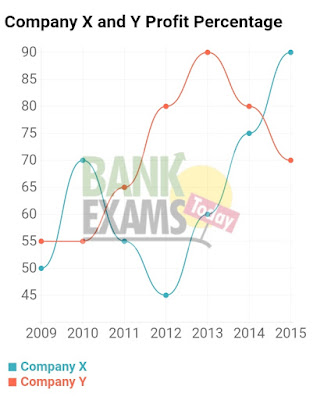New Students Offer - Use Code HELLO

# Expected Data Interpretation Set for IBPS PO Pre

### Study the following Line chart and the following questions carefully :Click The Image to Zoom
1. If the profit earned in 2011 by Company Y was Rs. 8,12,500, what was the total income of the Company in that year?

1.Rs. 12, 50, 000
2.Rs. 20, 62, 500
3.Rs. 16, 50, 000
4.Rs. 18, 25, 000
5.Rs. 17,78,000

2. If the amount invested by the two Companies in 2010 was equal, what the ratio between total income in 2010 of the Companies X and Y respectively?

1. 31 : 33
2.33 : 31
3. 34 : 31
4.14 : 11
5. None of these

3. If the total amount invested by the two Companies in 2014 was Rs. 27 lakhs, while the amount invested by Company Y was 50% of the amount invested by company X, what was the total profit earned by the two Companies together?

1.Rs. 21. 15 lakhs
2.Rs. 20. 70 lakhs
3.Rs. 18. 70 lakhs
4.Rs. 20. 15 lakhs
5.None of these

4. If the investments of Company X in 2012 and 2013 were equal. What is the difference between profit earned in two years if the income in 2013 was Rs. 24 lakhs?

1.Rs. 2. 25 lakhs
2.Rs. 3. 6 lakhs
3.Rs. 1. 8 lakhs
4.Rs. 2. 6 lakhs
5.none of these

5.If each of the Companies X and Y invested Rs. 25 lakhs in 2015, what was the average profit earned by the two companies?

1.Rs. 18 lakhs
2.Rs. 22. 5 lakhs
3.Rs. 17. 5 lakhs
4.Rs. 20 lakhs
5.none of these

1.2

Let expenditure =x
65% of x = 8,12,500
x=12,50,000
Income = Expenditure + Profit
= 12,50,000 + 8,12,500
= 20,62,500

2. 3

Let the expenditure of X= expenditure of Y= a
Income of X = 170% of a
= 1.7 a
Income of Y= 155% of a
= 1.55 a
Ratio = 1.7//1.55 = 34/31

3.2

Let the amount invested by Y =x
And amount invested by X=2x
x+2x=27
x=9
Expenditure of X=2x=18
Expenditure of Y=x=9
Profit earned by X= 75% of 18=13.5
Profit earned by Y= 80% of 9 = 7.2
Total profit earned by two companies = 20.7

4.1

Let the investment of X in 2012=x
And investment of X in 2013 =x
Income of X in 2013 =24
Income of X in 2013= 160% of x = 1.6x [ 60% Profit]
1.6 x=24
x =15
profit in 2012= 45% of 15=6.75
profit in 2013= 60% of 15 = 9
Difference = = 2.25

5.4

Investment of X in 2015 = 25
Profit of X in 2015 = 90% of 25 = 22.5
Investment of Y in 2015= 25
Profit of Y in 2015 = 70% of 25 = 17.5
Average profit =40/2=20